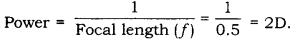# In what S.I. unit is the power of lenses stated? A convex lens has a focal length of 50 cm. Calculate its power

In what S.I. unit is the power of lenses stated? A convex lens has a focal length of 50 cm. Calculate its power.

The S.I. unit of power of a lens is dioptre.
We have, Focal length (f) = 50 cm = 0.5 m